# Non linear dynamics of a thin narrow ribbon in an airflow

## Alexandra Afanaseva1, Alexander Gouskov2, Grigory Panovko3

1, 2, 3Mechanical Engineering Research Institute of the Russian Academy of Sciences, Moscow, Russia

1Bauman Moscow State University, Moscow, Russia

1Corresponding author

Vibroengineering PROCEDIA, Vol. 32, 2020, p. 105-110. https://doi.org/10.21595/vp.2020.21359
Received 27 February 2020; accepted 5 March 2020; published 29 June 2020

46th International Conference on Vibroengineering in St. Petersburg, Russia, June 29 - July 1, 2020

Copyright © 2020 Alexandra Afanaseva, et al. This is an open access article distributed under the Creative Commons Attribution License, which permits unrestricted use, distribution, and reproduction in any medium, provided the original work is properly cited.
Views 79
Abstract.

The nonlinear aeroelastic vibrations of the Windbelt System are described in the paper. The system works based on aeroelastic flutter principle. The long narrow ribbon with pinned supports at the ends is used as mechanical model. The analysis of aerodynamic forces for a thin rectangular cross-section of the ribbon is provided. Lateral-torsional oscillations of the system is derived, considering aerodynamic forces. Conditions for generation of oscillations are determined using Poincaré-Andronov-Hopf bifurcation. The critical speed, which defines dynamic instability (aeroelastic flutter), is determined as well. The influence of the tension force and other ribbon parameters on the critical speed is considered. The supercritical behavior of the system is investigated.

Highlights
• The mathematical model for the lateral-torsional oscillations of a flexible ribbon under the influence of oncoming air flow is derived and analyzed.
• They describe the dynamics of a Wind belt type wind generator in a simplified form.
• Small lateral-torsional oscillations of the system are analyzed.
• The value of the critical velocity, the trajectory of motion, and the bifurcation diagram are obtained.

Keywords: wind power, Windbelt, aeroelastic oscillations, flutter, self-oscillations.

#### 1. Introduction

Wind energy is one of the main areas of alternative energy. It is based on conversion of kinetic energy of the air masses into electrical, mechanical or thermal power. The transformation of airflow energy into electrical energy is usually accomplished through wind turbines. Recently, the possibility of producing electric energy using a wind generator, called “Wind Belt” [1-5], is being intensively studied.

The schematic diagram of the device is shown in Fig. 1; taken from . A permanent magnet 3 is fixed on a flexible tape 2 stretched between two rigid supports 1 (constructions with two or more magnets are also known ). Inductance coils 5 are arranged coaxially with the magnet on top and bottom of the ribbon on the frame 4. The airflow can disturb the static balance of the ribbon and lead to the excitation of its oscillation as an aeroelastic flutter  under certain conditions. The vibrating movement of the magnets induces current in nearby pickup coils, which subsequently can be rectified to a direct voltage.

Fig. 1. Schematic diagram of a wind generator “Windbelt”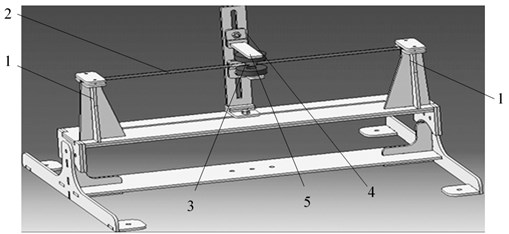There are a few studies related to the generation of electric current in such devices in the existing scientific literature. However, investigation of the oscillations of a thin ribbon depending on its tension and the airspeed has been studied insufficiently. Known computational models are based on various simplified assumptions that limit investigation of supercritical behavior based on bifurcation analysis.

The purpose of this work is to model the dynamics of the ribbon in the air flow, analyze its movement and the possibility of structure optimization.

#### 1.1. Problem statement

A ribbon of length $l$ with hinged supports on both ends is positioned in the oncoming air flow (Fig. 2). It is stretched by the longitudinal force $T$ along the $Z$ axis. The cross section of the ribbon is constant along the length. The ribbon in horizontal air flow of constant rate $U$ has lifting force (wind load), which is evenly distributed along the length.

The net lifting force is applied along a line that passes through the pressure centers of cross section. This line is parallel to the $Z$-axis at a distance ${x}_{0}=b/4$ (Fig. 2).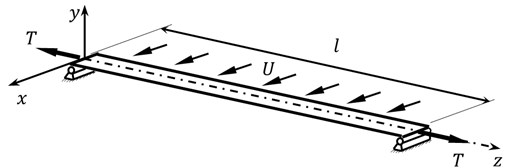a)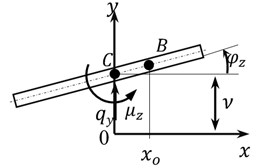b)

The lifting force ${q}_{y}$ is distributed over length and applied to the center of pressure line (point $B$ in Fig. 2) ${q}_{y}=\left(\rho {U}^{2}/2\right)b{C}_{y}$ , where $\rho$ is the density of the environment, $b$ is the width of the cross section of the ribbon, ${C}_{y}$ – is lifting force coefficient. The lifting force point $B$ is relocated to the center of the cross section, point $C$. To compensate this transition, the force system is supplemented by linear torques ${\mu }_{z}={C}_{y}\rho {U}^{2}{b}^{2}/8$.

The lifting force coefficient ${C}_{y}$ for a thin rectangular profile of the ribbon is given by a known solution for a flow of an ideal incompressible fluid ${C}_{y}=2\pi \mathrm{s}\mathrm{i}\mathrm{n}\left({\stackrel{~}{\phi }}_{z}\right)\approx {A}_{1}\left({\stackrel{~}{\phi }}_{z}-{A}_{3}{\stackrel{~}{\phi }}_{z}^{3}\right)$, where ${A}_{1}=2\pi$, ${A}_{3}=2\pi /6$ are the expansion coefficients, ${\stackrel{~}{\phi }}_{z}={\phi }_{z}-\left({\stackrel{˙}{\phi }}_{z}b/4+\stackrel{˙}{v}\right)/U$ is the effective angle of attack, $v$ is the vertical movement of the ribbon axis (pole).

#### 1.2. Equations of motion

The motion of the considered model is described by a system of equations for lateral-torsional oscillations of a string on hinged supports, which is loaded with distributed force and distributed moment . Taking into account the relocation of lifting forces to the center of gravity and the expression for the lifting coefficient, the system becomes:

(1)

where ${\rho }_{1}$ is the density of the ribbon, $G$ is the shear modulus, $A=bh$ is area of cross section, ${I}_{0}=bh\left({b}^{2}+{h}^{2}\right)/12$ is the polar moment of inertia, ${I}_{k}=b{h}^{3}/3$ – is geometric stiffness of the section (strip) during torsion, ${d}_{v}$, ${d}_{\phi }$ – is linear viscous Rayleigh damping coefficients in bending and torsion, respectively.

Further, Eq. (1) is reduced to a dimensionless form. Whereupon, dimensionless parameters are introduced: time $\tau =t/{t}^{\mathrm{*}}$, deflection $\xi =v/{V}^{\mathrm{*}}$, coordinate $\zeta =z/{Z}^{\mathrm{*}}$, velocity $\mathrm{\Lambda }=U/{U}^{\mathrm{*}}$, force $\theta =T/{T}^{\mathrm{*}}$, and scales ${t}^{\mathrm{*}}=l/h\sqrt{{\rho }_{1}\left({b}^{2}+{h}^{2}\right)/4G}$, ${U}^{\mathrm{*}}=\sqrt{8G{h}^{3}/\left(3{\rho }_{0}{a}_{0}b{l}^{2}\right)}$, ${V}^{\mathrm{*}}=h$, ${Z}^{\mathrm{*}}=l$, ${T}^{\mathrm{*}}=G{h}^{2}$. The systems of Eq. (1) take the form:

(2)

All of them are expressed in terms of two fixed dimensionless parameters $\epsilon =h/b$ and $\kappa =\sqrt{\rho /{\rho }_{1}}$:

Damping coefficients are equal to 5 % of its critical value ($k$ is mode number).

#### 1.3. The calculation of the critical speed

The system of Eq. (2) is reduced to a system with a finite number of degrees of freedom using the Galerkin method. The solution is represented in the form of an expansion on the basis where ${u}_{k}\left(\zeta \right)={C}_{k}\mathrm{s}\mathrm{i}\mathrm{n}\left(k\pi \zeta \right)$ are functions satisfying the boundary conditions for the considered model (Fig. 2). The constant ${C}_{k}$ is determined from the condition of basis property and orthonormality of functions ${u}_{k}\left(\zeta \right)$.

After applying the Ritz-Galerkin method Eq. (2) will take the form:

(3)

where $B=\underset{0}{\overset{1}{\int }}{u}_{k}^{4}d\zeta$.

The existing trivial solution of the Eq. (3) corresponds to the equilibrium position, which is defined as an unperturbed motion. The Eq. (3) is linearized near the equilibrium position for stability analysis. Then, the Eq. (3) in matrix form:

(4)
$\mathbf{M}\stackrel{¨}{\mathbf{x}}+\mathbf{D}\stackrel{˙}{\mathbf{x}}+\mathbf{C}\mathbf{x}=0,$
.

Solutions of the Eq. (4) are sought in the form $\mathbf{x}={e}^{\lambda \tau }\mathbf{u}$. Then, the characteristic equation to determine $\lambda$ takes the form $\mathrm{d}\mathrm{e}\mathrm{t}\left({\lambda }^{2}\mathbf{M}+\lambda \mathbf{D}+\mathbf{C}\right)=0$. Further, the solution is considered only for the first mode (1) with the following parameter values $\epsilon =$ 0.002, $\kappa =$ 0.03.

The dimensionless tension force $\theta$ is set in a way that the dynamic loss of stability occurs earlier than the static one (divergence). Otherwise, there is a divergence phenomenon when torsional rigidity of the ribbon is completely lost.

Fig. 3(a) shows the dependence of the critical velocity ${\mathrm{\Lambda }}_{\mathrm{*}}$ on the tension force $\theta$. When a certain value of the tension force ${\theta }_{\mathrm{*}}$ is reached, the critical speed becomes equal to $\pi$ and does not grow with further increase of $\theta$. This value of the critical velocity ${\mathrm{\Lambda }}_{\mathrm{*}}$ corresponds to divergence. This is confirmed by Fig. 3(b) and Fig. 3(c) when $\theta >{\theta }_{\mathrm{*}}$ (○ – beginning of trajectory, × – trajectory end). The first trajectory intersects the $\mathrm{R}\mathrm{e}\left(\lambda \right)=0$ axis at in the value ${\mathrm{\Lambda }}_{\mathrm{*}}=\pi$ (Fig. 3(b)) that corresponds to the transition of the same trajectory through the origin at the Argand diagram (Fig. 3(c)). It corresponds to static loss of stability.

Fig. 3. a) The dependence of the critical speed ${\mathrm{\Lambda }}_{\mathrm{*}}$ on the tension force $\theta$; b) the dependence $\mathrm{R}\mathrm{e}\left(\lambda \right)$on $\mathrm{\Lambda }$; c) static stability loss on the Argand diagram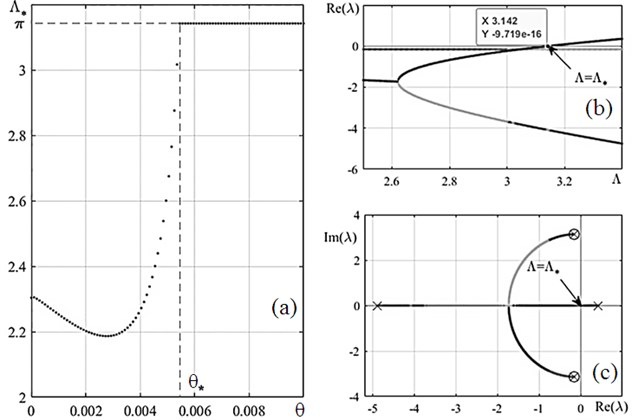An analysis of the results shows that the parameter $\theta$ must be in the range of $\left(0,{\theta }_{\mathrm{*}}\right)$ in order to observe dynamic loss of stability before static one. Here, it is defined as $\theta =$ 0.002. Fig. 4(a) shows trajectories of roots $\lambda$ for selected parameters. The point of their transition to the right half-plane is determined. The system has a critical velocity value ${\mathrm{\Lambda }}_{\mathrm{*}}$ at this point.

The value of the imaginary part has the meaning of the oscillation frequency ${\omega }_{f}$ of the system at $\mathrm{\Lambda }={\mathrm{\Lambda }}_{\mathrm{*}}$. The value of ${\omega }_{f}$ corresponds to the ordinate of the marked point $\mathrm{\Lambda }={\mathrm{\Lambda }}_{\mathrm{*}}$ in Fig. 4(a). The value of ${\mathrm{\Lambda }}_{\mathrm{*}}$ is defined by dependence of the real part of the roots $\mathrm{R}\mathrm{e}\left(\lambda \right)$ on $\mathrm{\Lambda }$ (Fig. 4(b)), at the point $\mathrm{R}\mathrm{e}\left(\lambda \right)$ equals zero ${\mathrm{\Lambda }}_{\mathrm{*}}=$ 2.203.

Fig. 4. a) The Argand diagram and b) dependence of the real part of the roots $\lambda$ on the dimensionless velocity $\mathrm{\Lambda }$; ○ – beginning of trajectory, × – trajectory end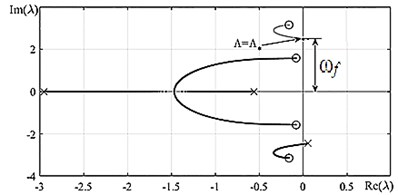a)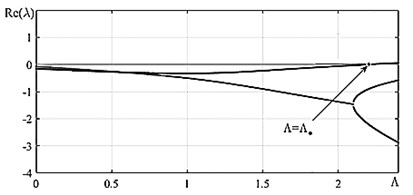b)

#### 1.4. Analysis of supercritical behavior

To study supercritical behavior the nonlinear system (see Eq. (3)) is integrated. A speed value $\mathrm{\Lambda }$ greater than ${\mathrm{\Lambda }}_{\mathrm{*}}$ by 10 % is used. The motion is considered at the dimensionless time interval $\tau =30{T}_{f}$, where ${T}_{f}={1/\omega }_{f}$ is the wave number for the frequency ${\omega }_{f}$.

Numerical simulation of the Eq. (3) shows that periodic solutions can be established, i.e., there are both unstable equilibrium position and stable periodic motions in the supercritical region.

To evaluate the establishment of a self-oscillatory regime, phase portraits in Fig. 5(a) and Fig. 5(b) of vertical dimensionless movement $\xi \left(\tau \right)$ and dimensionless rotation of the cross-section $\phi \left(\tau \right)$ from the Eq. (3) are derived for different initial conditions: 1) $\left\{\xi ,\stackrel{˙}{\xi },\phi ,\stackrel{˙}{\phi }\right\}=\left\{0,0.85,0,0\right\}$, 2) $\left\{\xi ,\stackrel{˙}{\xi },\phi ,\stackrel{˙}{\phi }\right\}=\left\{130,0,0,0\right\}$.

The attractions of the trajectories to the limit cycles are clearly visible in Fig. 5.

Fig. 5. Phase portraits for coordinate functions at a) 1/2 lateral oscillations $\left\{\stackrel{˙}{\xi },\xi \right\}$ and b) torsional oscillations ; “ –– “ – trajectory with initial conditions No. 1; “– – –“ – trajectory with initial conditions No. 2; ○ – beginning of trajectory, × – trajectory end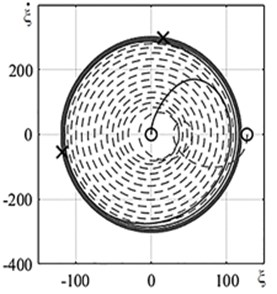a)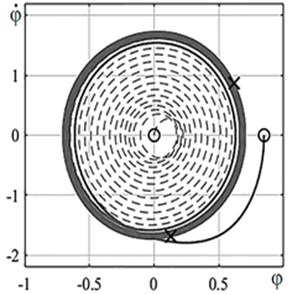b)

The analysis of stability of the equilibrium rectilinear position of the tape allows to plot a bifurcation diagram (Fig. 6). It was shown above that there are stable periodic motions in the supercritical, region that corresponds to a stable branch on the bifurcation diagram. Numerical integration by establishing method is utilized to plot the diagram, . The results for the amplitudes ${A}_{\xi }$ and ${A}_{\phi }$ are presented in Fig. 6(a) and Fig. 6(b), respectively.

Fig. 7. The bifurcation diagram a) for $\xi$ and b) for $\phi$: ● – stable motion, × – unstable motion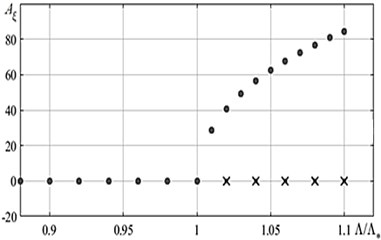a)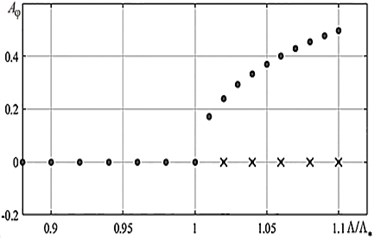b)

#### 2. Conclusions

The mathematical model for the lateral-torsional oscillations of a flexible ribbon under the influence of oncoming air flow is derived and analyzed. The value of the critical velocity, the trajectory of motion, and the bifurcation diagram are obtained. Small lateral-torsional oscillations of the system are analyzed. They describe the dynamics of a Windbelt-type wind generator in a simplified form. The calculations show that there is a hyperbolic law.

#### Acknowledgements

The work is supported by the Russian Science Foundation (project No. 18-19-00708).

1. Frayne S. Generator Utilizing Fluid Induced Oscillations. Patent US7573143 B2, 2009. [Search CrossRef]
2. Windbelt Cheap Micro Wind Generator, http://www.reuk.co.uk/wordpress/wind/windbelt-cheap-micro-wind-generator/. [Search CrossRef]
3. Vu Dinh Quy, Nguyen van Sy, Dinh Tan Hung, Vu Quoc Huy Wind tunnel and initial field tests of a micro generator powered by fluid-induced flutter. Energy for Sustainable Development, Vol. 33, 2016, p. 75-83. [Publisher]
4. Bibo A., Li G., Daqaq M. F. Electromechanical modeling and normal form analysis of an aeroelastic micro power generator. Journal of Intelligent Material Systems and Structure, Vol. 22, Issue 6, 2011, p. 577-592. [Publisher]
5. Bryant M., Garcia E. Modeling and testing of a novel aeroelastic flutter energy harvester. Journal of Vibration and Acoustics, Vol. 133, Issue 1, 2011, p. 011010. [Publisher]
6. Fung Y. C. An Introduction to the Theory of Aeroelasticity. Wiley, New York, 2008. [Search CrossRef]
7. Vibrations in Tehnique, Vol. 1: Linear Systems Oscillations. Moscow, Mashinostroenie Publ, 1978, p. 352, (in Russian). [Search CrossRef]
8. Nayfeh A. H., Mook D. T. Nonlinear Oscillations. Wiley, New York, 1979. [Search CrossRef]

#### Cited By

 NODYCON Conference Proceedings Series Janis Viba, Grigory Panovko, Alexander Gouskov, Martins Irbe 2022 Journal of Machinery Manufacture and Reliability A. A. Afanaseva, A. M. Gouskov, G. Ya. Panovko 2021